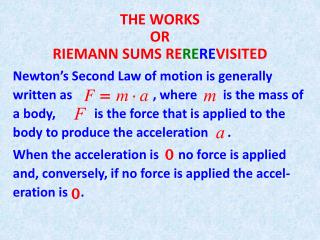DownloadDownload PresentationTHE WORKS OR RIEMANN SUMS RE RE RE VISITED

# THE WORKS OR RIEMANN SUMS RE RE RE VISITED

Télécharger la présentation## THE WORKS OR RIEMANN SUMS RE RE RE VISITED

- - - - - - - - - - - - - - - - - - - - - - - - - - - E N D - - - - - - - - - - - - - - - - - - - - - - - - - - -
##### Presentation Transcript

1. THE WORKSORRIEMANN SUMS REREREVISITED Newton’s Second Law of motion is generally written as , where is the mass of a body, is the force that is applied to the body to produce the acceleration . When the acceleration is no force is applied and, conversely, if no force is applied the accel-eration is .

2. When one does Physics, or Chemistry, or any other of the more applied sciences, one sticking point that always arises is the decision on what units to use to measure whatever we are interested in. Newton’s second law of motion is a nice case in point: In order to simplify calculations we would like to have , in other words the unit of force we choose should correspond to (one unit of mass)x(one unit of acceleration) In the SI metric system the unit of mass chosen is the kilogram (kg), and the unit of acceleration is

3. (longhand) (abbreviated) The resulting unit of force ( ) is called one (Choices of units are arbitrary, one could measure distances with any fixed rod, but they should be universally agreed upon, so scientists can commu-nicate!) In the British system Newton.

4. In order to avoid difficulties with “changes of direction”, that would force us to consider centripetal and centrifugal forces, we will limit ourselves to consider motion along a straight line. The physical definition of work is well known, workW (done by a force) is defined as W = (force)x(distance traveled) WARNING: forces in the same direction of motion are taken as positive in the formula, otherwise negative. Naturally, in the SI metric system, the unit of work is (one Newton)x(one meter) (one Joule J)

5. The work done by gravity when you drop a 5 kg stone from a height of is (recall that gravity is ) Computations with constant forces are easy, just multiply by the displacement (if you lift a stone some height and then lower it back to the ground, the displacement is , and that is the work you (or gravity) have done ! What if the force varies with the position ?

6. Can we compute the work? By now it’s old hat for us! Let represent the position, the force, . The work performed by as the position moves from to is So the total work performed by the force is the limit of the Riemann sums Of course, we compute the work as

7. Now we do examples. We start with the study of SPRINGS. They look like and there is a law, known as Hookes Law, that says that, if denotes the distance from the end of the spring to the position of that end when the spring is unstressed, then the spring reacts with a force where is a constant.

8. There are two kinds of possible problems: • are given (as a nifty word problem) and you are asked to compute • are given (as a nifty word problem), you are asked to compute , then to compute Let’s do both kinds. The first kind is trivial, if you know the integral is easy to compute.

9. As an example of the second kind, suppose you are told that a certain spring has unstressed length and that the force required to compress the spring down to is . How much work is needed to extend the spring from unstressed length to ? Solution: From Hookes Law we get (careful with the units!). Therefore And the needed force is

10. HANGING ROPES We are going to compute the work required to lift a weight with a rope that hangs down and weighs .

11. The work used up by is trivial, it’s What about the rope itself? Looking at the figure We see that the length (the blue piece) at distance from the end of the rope (not necessarily the ground!) has to be lifted for

12. and weighs The work required to lift up the blue piece is therefore . The total work used to lift the rope (just the rope !) is (based on our experience with Riemann sums) (Where did the come from?) What if the rope gets heavier the further away from the end, say ?

13. So now we have The prevous formula for the work on the rope becomes which changes the integral to Now do lots of homework!